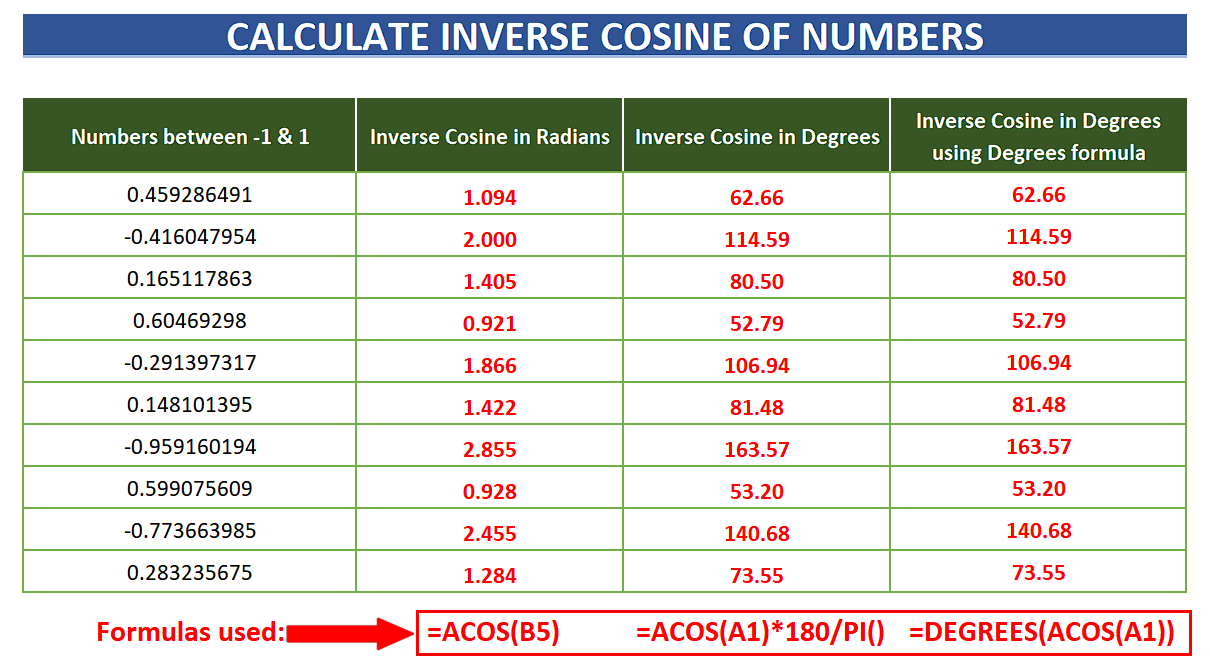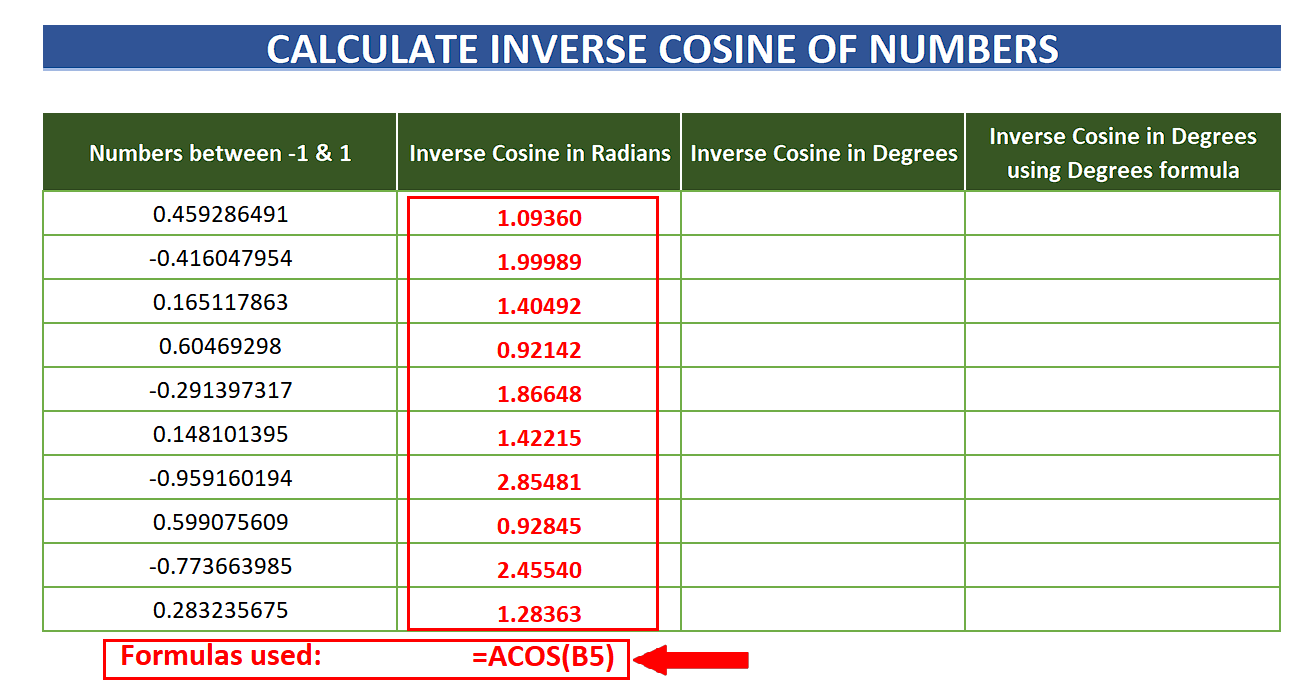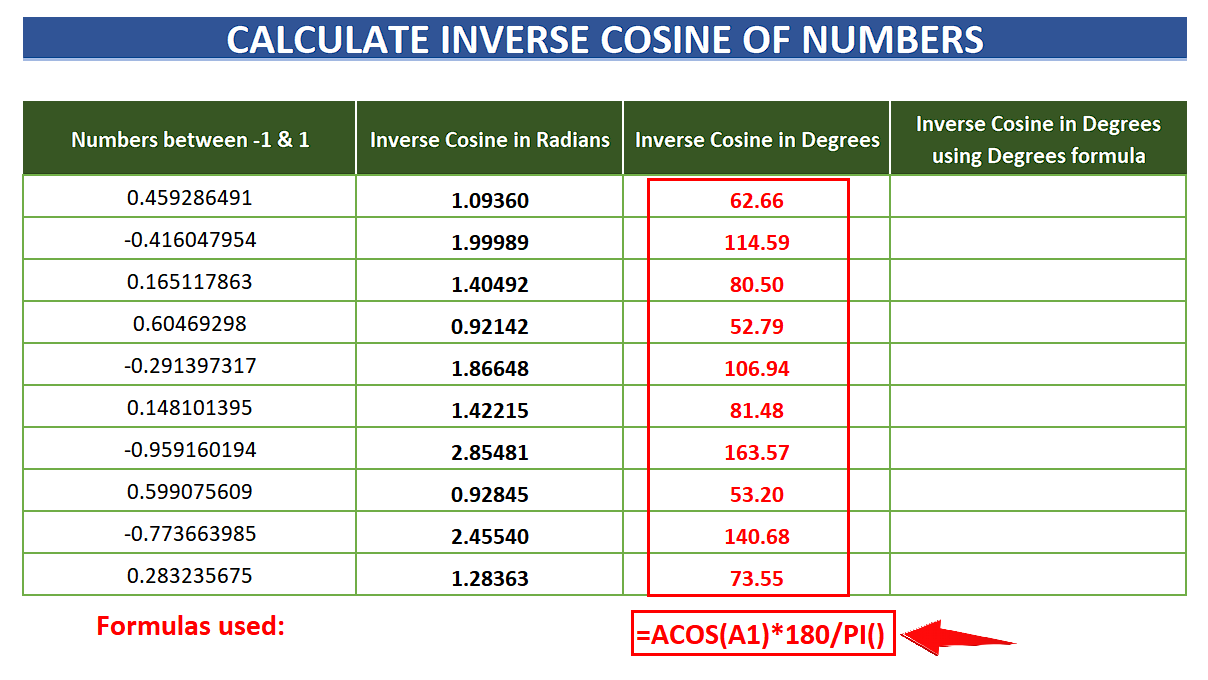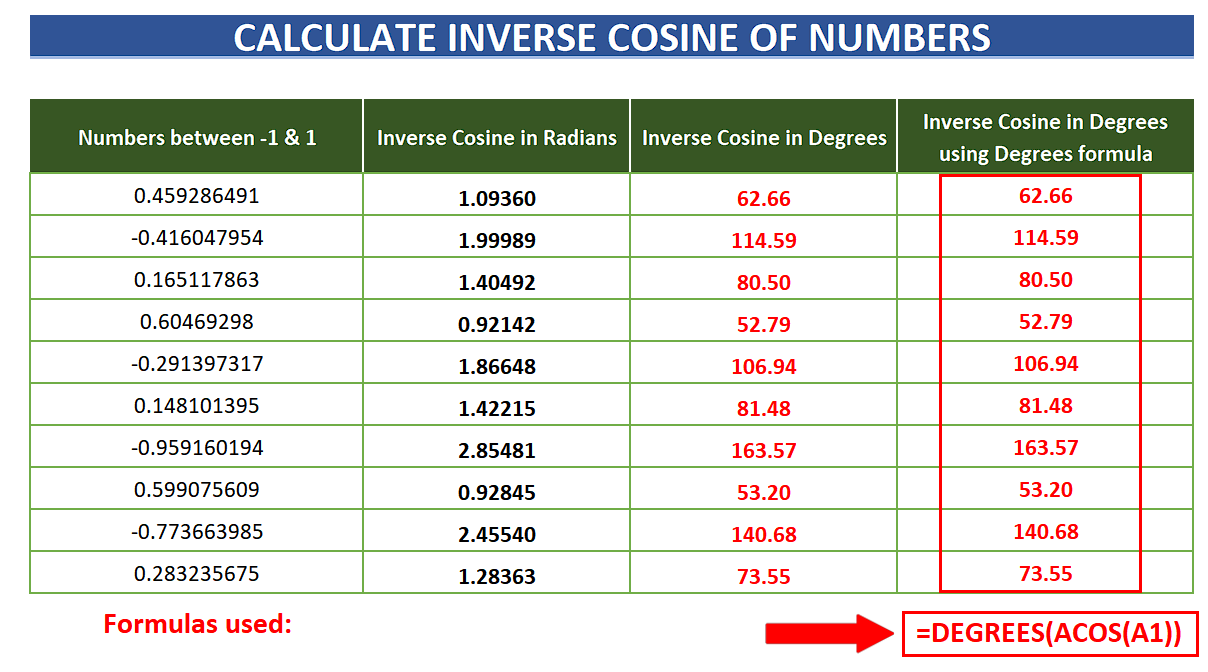# How to find the inverse cos of numbers in Excel

In today’s tutorial we’ll learn how to calculate inverse cosines of numbers. However, the numbers must be in between -1 to 1 which is the range of the COSINE function. If the numbers are not in the above mentioned range, then the formula will produce errors. Let’s look at the dataset where we have the numbers between -1 and 1. We’ll find the INVERSE COSINE of these numbers by the following methods;

• Using ACOS to get results in Radians
• Using ACOS with 180/pi to get the results in Degrees
• Using ACOS with DEGREES function to get the results in DegreesExcel is a very powerful tool for data analysis and performing all sorts of calculations. While working with the Geometrical data we need to calculate the triangular ratios or the inverse of the triangular ratios as well. In this scenario Excel provides built-in functions to calculate both regular ratios and inverse ratios of a triangle.

### Step 1 – Calculate ACOS to get values in Radians– The first method is to simply use the ACOS function. Inverse cosine can be calculated by using this formula mentioned below;
=ACOS(A1)

– This will return the values of angles in Radians. To implement the formula to the whole column just double click the fill handle and the formula will be implemented on the whole column values.

### Step 2 – Calculate ACOS to get values in Degrees– The method to calculate inverse cosine of numbers and to get results in degrees is to simply multiply the values returned by ACOS with 180/pi(). This will convert the values in Radians to Degrees. Using the below mentioned formula Inverse cosine can be calculated in degrees;
=ACOS(A1) * 180/pi()

– This will return the values of angles in degrees. To implement the formula to the whole column just double click the fill handle and the formula will be implemented on the whole column values.

### Step 3 – Calculate ACOS to get values in Degrees by using DEGREES function– The method to calculate the inverse cosine of numbers and to get results in degrees is to use ACOS inside the DEGREES function. Using the below mentioned formula Inverse cosine can be calculated in degrees;
=DEGREES(ACOS(A1))

– This will return the values of angles in degrees. To implement the formula to the whole column just double click the fill handle and the formula will be implemented on the whole column values.